## How to write greek letters along with english lett...

Greetings,

help

i want to add text with greek letters and english letters on top of the graph with out using title in plot

restart:
with(plots):
p1:=plot(sin(2*x), x = -Pi .. Pi):
p2:= textplot(effect of alpha =3,gamma=5 in the flow):
display(p1,p2):

## why pdsolve only solves this wave PDE when telling...

This is a Wave PDE inside disk. with ﬁxed edge of disk,  and no theta dependency. initial position and velocity  given.

When using "c^2" for the  wave propagation speed, Maple only gives solution when also using assumptions to tell that c is positive.

```restart;
Physics:-Version();
`2018, November 28, 1:35 hours, version in the MapleCloud: 224, version installed in this computer: 224````

And the PDE is

```pde := diff(u(r, t), t\$2) = c^2*( diff(u(r,t), r\$2)  + (1/r)* diff(u(r,t),r)  ) ;
ic:=u(r,0)=1, eval( diff(u(r,t),t),t=0)=r/3;
bc:=u(1,t)=0;
sol:=pdsolve([pde, ic,bc], u(r, t)) assuming t>0;
```

sol:=()

Now adding assuming c>0, or "c::positive", or "c>=0", it solves it

```restart;
pde := diff(u(r, t), t\$2) = c^2*( diff(u(r,t), r\$2)  + (1/r)* diff(u(r,t),r)  ) ;
ic:=u(r,0)=1, eval( diff(u(r,t),t),t=0)=r/3;
bc:=u(1,t)=0;
sol:=pdsolve([pde, ic,bc], u(r, t)) assuming t>0,c>=0;
```

Any idea why the assumption "c>0"  is needed when the speed is given as "c^2" ? I also tried assumption "c::real", but it still did not solve it.   It seems related to Maple using Laplace transform to solve it.

It seems to me the assumption c>0 should not needed here. But if it is, I'd like to learn why.

Maple 2018.2, windows 10

Update

Thanks to comment below

" so u(r, 0)=1 (for all r), and u(1, t)=0 (for all t). So what is u(1,0)??? "

I should have added "r>0,r<1".  The reason I did not, is that I copied the code to solve this from Mathematica. In Mathematica, it did not need this assumption to solve it. It seems to depend on the method of solution used by Mathematica vs. Maple to cause this difference.

But now I see it helps to have it there. With the above assumption, now, there is no need to do any assumption on "c" at all and it gives solution. adding c>0 or t>0 or c>=0, makes no change now.

```restart;
pde := diff(u(r, t), t\$2) = c^2*( diff(u(r,t), r\$2)  + (1/r)* diff(u(r,t),r)  ) ;
ic:=u(r,0)=1, eval( diff(u(r,t),t),t=0)=r/3;
bc:=u(1,t)=0;
sol:=pdsolve([pde, ic,bc], u(r, t)) assuming t>0,r>0,r<1;
```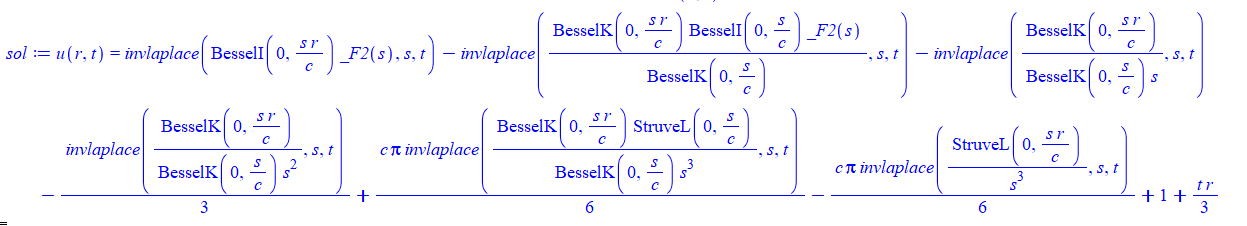## Pls help me find the law...

Hi

I am trying to find how it calculates how much solar will be exported back to the grid. I thought this could only be estimated  empirically, but apparently not.

Anyway, it depends on three factors, power consumption, power installed, and average hours of sun per day, or hours per day as a function of time of year. For C=4.5kWh/day and S=5kW and H=4 sun hrs per day, means 90% will be exported back to the grid Y. So Y(C,S,H)= a closed form expression.

How to guess the fuction? I tried array interpolation for a numeric solution, but it complains: independent coordinates must be sorted in increasing order

law.mw## ordinary differential equation...

When you are solving an ode of degree 4 in maple you get an answer like this : f(x)=aZ1(x)+ bZ2(x)+ cZ3(x) + dZ4(x) Now I want to pick Z1 and Z2 and others one by one to form a list. What should I do ? (I have tried to use coeff but it did not work)

## How do I have a vector exponential, and integrate ...

Hello, I have two main questions involving the attached file.

1. In the first error, I am trying to put a vector in the exponential, however I am getting an error. Is there any way around this?

2. I am then trying to integrate an OuterProductMatrix, however I am getting another error. Is there another way around this?

Thank you.

## Another way to do `union`...

Is there another way in maple to code `union`. I am not confident using this function and was wondering if there was a perhaps longer/different way to do the same thing?

Thanks!

## Solve inequality system whose variable is indexed ...

I have the following inequality system :

[ U1,2 + U1,3 -1 = 0,

U2,3 - U1,2 = 0,

-U1,3 -U2,3+1 = 0,

0 <=U1,2,

0 <= U1,3,

0 <= U2,3]

I want to solve it using LinearMultivariateSystem, namely I excuted a command :

Then the following is return :

`Error, (in Utilities:-SimpleAnd) invalid input: a string/name list is expected for sort method `lexorder``

I tried that

My question is that how do you use LinearMultivariateSystem for varibles indexed by two params.

Thank you.

## Select elements of list wrt criteria...

Hiya

from this

>with(combinat):
>A:=choose([CPC__h, SIZE__h,CPC__m, SIZE__m,CPC__l, SIZE__l],2):

where h denotes high, m=medium, l=low

I want to select CPC and SIZE all the combinations w.r.t h, m and l

[[CPC__h, SIZE__h], [CPC__h, SIZE__l], [CPC__h, SIZE__m], [CPC__l, SIZE__h], [CPC__l, SIZE__l], [CPC__l, SIZE__m], [CPC__m, SIZE__h], [CPC__m, SIZE__l], [CPC__m, SIZE__m]]

>nops(A)-3

9

I want to apply the methodology to
choose([CPC__h, SIZE__h, SH__h,CPC__m, SIZE__m, SH__m,CPC__l, SIZE__l, SH__l],3)

## I have a question...

hi , I want to solve a problem of structural analysis (slope deflection) in maple but .... ?## why convert(..,Int) does not work for invlaplace?...

convert(...,Int) in Maple 2018.2 works for fourier, invfourier, laplace, but does not work for invlaplace.

Why is that? Is there a workaround?

```expr:=fourier(f(x), x, w):
convert(expr,Int);
```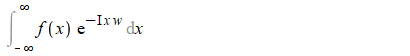```expr:=invfourier(f(w), w, x):
convert(expr,Int);
```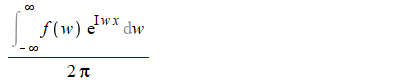```expr:=laplace(f(s),s,t):
convert(expr,Int)
```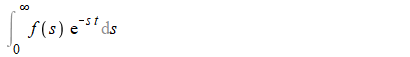```expr:=invlaplace(f(s),s,t):
convert(expr,Int)
```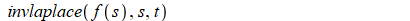Was expecting to see the Mellin's inverse formula.

Maple 2018.2 on windows 10

## How to read Help on Eigenvectors...

In the snip from Maple help: please expaln (a) the meaning, (b) how do I enter e1..-1,2 in the last statement?

Thanks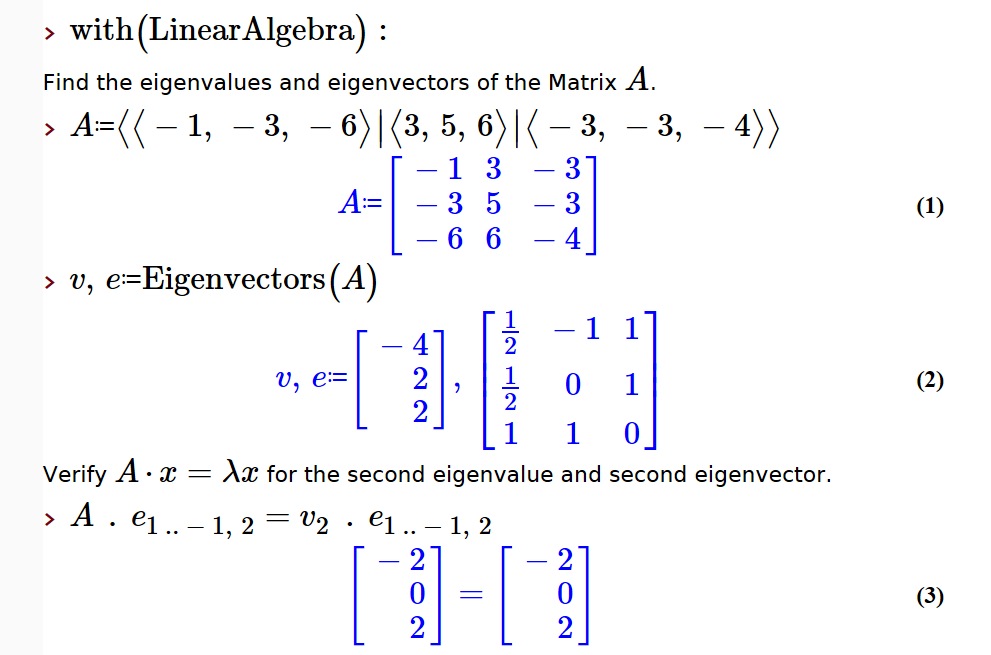## Error, (in dsolve/numeric/bvp) numeric exception: ...

Hi I am trying to solve the following system of equation. I could solve it for approximate value of s(0)=0.9999 using middefer method of bvp, but as soon as I give the b.c. as s(0)=1, Maple gives me an error that there is an numeric exception. Can someone explain it what is going wrong with s(0)=1? Any sugesstion to solve the equation?
error.mw

b := 1;
r := .1;
l := 3;
a := 10; p := 1.5; ds := 100; dk := 1;

sol3 := dsolve({ds*(diff(i(x), x)) = exp(eta(x)), s(x)^3*i(x)*b*r+(1-s(x))^3*a*l*(diff(s(x), x))/s(x)^1.5 = (1-s(x))^3, diff(eta(x), x) = dk*(i(x)-1)/s(x)^p, i(0) = 0, i(1) = 1, s(0) = 1}, {eta(x), i(x), s(x)}, approxsoln = [eta(x) = .5, i(x) = .5, s(x) = 1], initmesh = 100000, type = numeric, maxmesh = 100000, range = 0 .. 1, output = listprocedure, method = bvp[middefer], abserr = 0.1e-3, adaptive = true);

Error, (in dsolve/numeric/bvp) numeric exception: division by zero

plots[odeplot](sol3, [[x, s(x)]], 0 .. 1, color = ["Green"]);

Thanks and regards,

## thanks my problem solved by Maple experts thanks m...

write a programe for fractional calculus and it shows subscript error plzz help me with that## pdsolve gives PDEtools/eval/2) numeric exception:...

Using Latest Physics updates (I am not sure when this started), pdsolve gives Error, (in PDEtools/eval/2) numeric exception: division by zero on the following problem from a HW from text book.

```restart;
"220"

pde:=diff(w(x,t),t)+3*t*diff(w(x,t),x)=w(x,t);
ic:=w(x,0)=f(x);
sol:=pdsolve([pde,ic],w(x,t));
```

Error, (in PDEtools/eval/2) numeric exception: division by zero

```pde = D[w[x, t], t] + 3 t D[w[x, t], x] == w[x, t];
ic = w[x, 0] == f[x];
sol = Simplify[DSolve[{pde, ic}, w[x, t], {x, t}]]```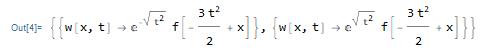This is on Maple 2018.2 on windows 10 64 bit.

Any idea what is causing this and any workaround? Do others get the same exception?